# Graph Classification Tutorial¶

Author: Mufei Li, Minjie Wang, Zheng Zhang.

In this tutorial, you learn how to use DGL to batch multiple graphs of variable size and shape. The tutorial also demonstrates training a graph neural network for a simple graph classification task.

Graph classification is an important problem with applications across many fields, such as bioinformatics, chemoinformatics, social network analysis, urban computing, and cybersecurity. Applying graph neural networks to this problem has been a popular approach recently. This can be seen in the following reserach references: Ying et al., 2018, Cangea et al., 2018, Knyazev et al., 2018, Bianchi et al., 2019, Liao et al., 2019, Gao et al., 2019).

In this tutorial, you learn how to perform batched graph classification with DGL. The example task objective is to classify eight types of topologies shown here.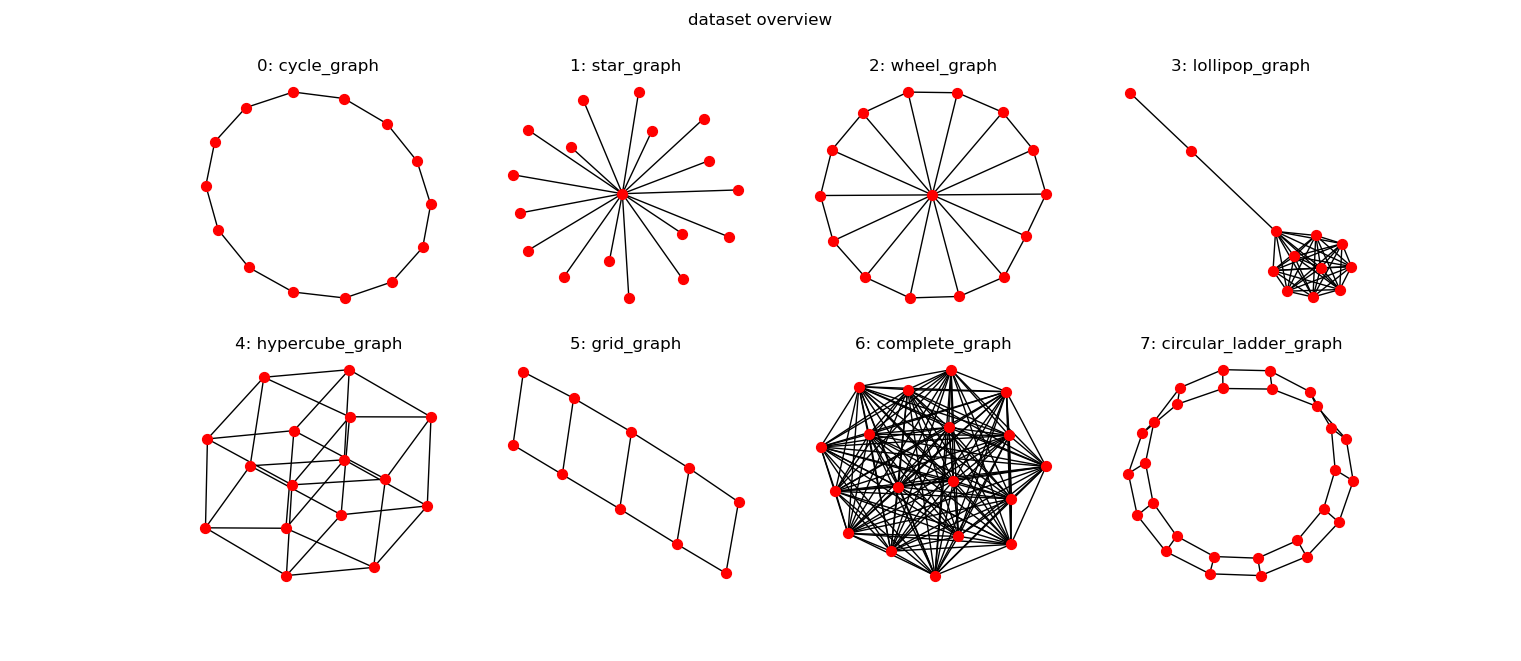Implement a synthetic dataset data.MiniGCDataset in DGL. The dataset has eight different types of graphs and each class has the same number of graph samples.

import dgl
import torch
from dgl.data import MiniGCDataset
import matplotlib.pyplot as plt
import networkx as nx
# A dataset with 80 samples, each graph is
# of size [10, 20]
dataset = MiniGCDataset(80, 10, 20)
graph, label = dataset
fig, ax = plt.subplots()
nx.draw(graph.to_networkx(), ax=ax)
ax.set_title('Class: {:d}'.format(label))
plt.show()## Form a graph mini-batch¶

To train neural networks efficiently, a common practice is to batch multiple samples together to form a mini-batch. Batching fixed-shaped tensor inputs is common. For example, batching two images of size 28 x 28 gives a tensor of shape 2 x 28 x 28. By contrast, batching graph inputs has two challenges:

• Graphs are sparse.

• Graphs can have various length. For example, number of nodes and edges.

To address this, DGL provides a dgl.batch() API. It leverages the idea that a batch of graphs can be viewed as a large graph that has many disjointed connected components. Below is a visualization that gives the general idea.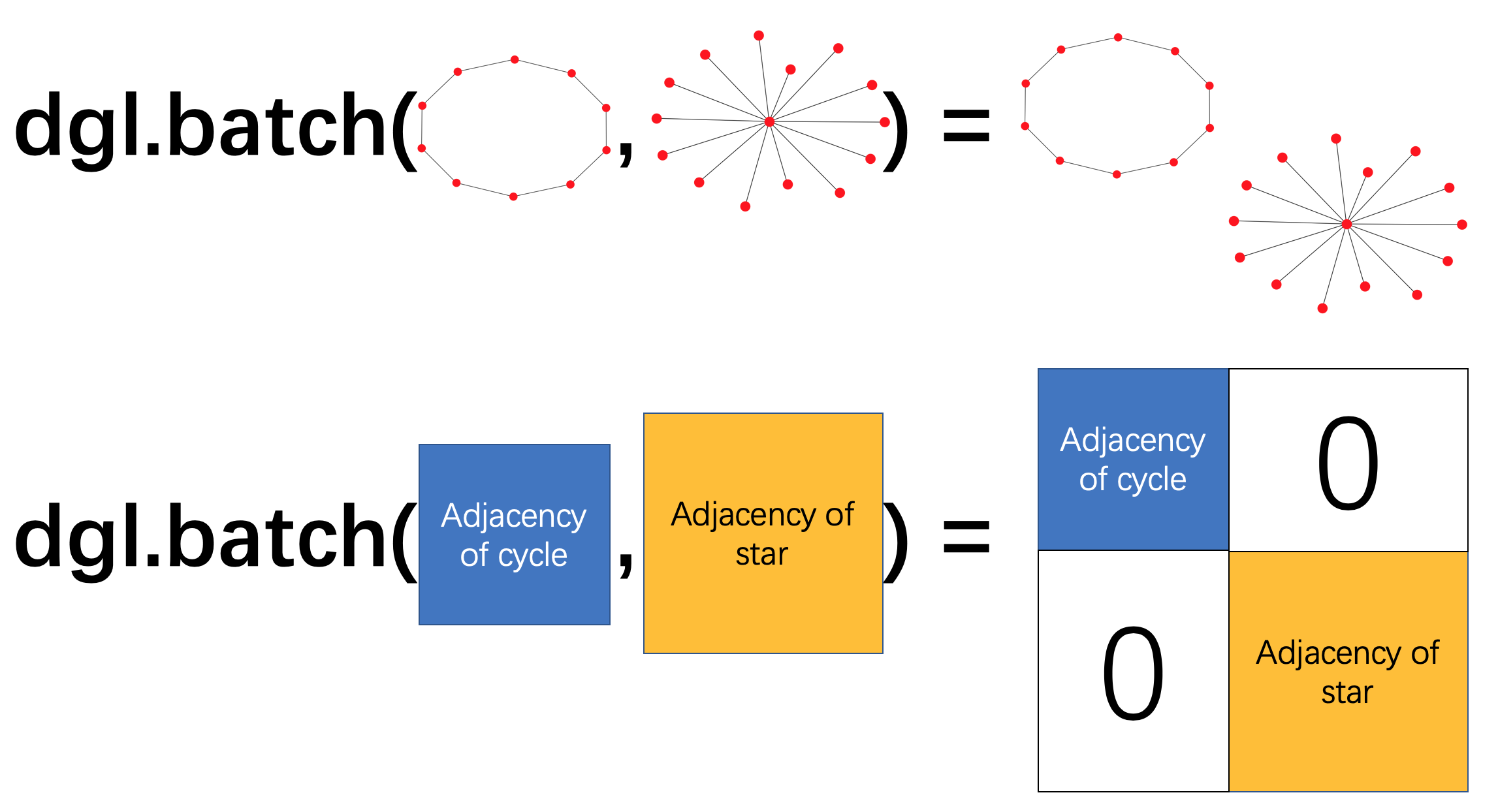The return type of dgl.batch() is still a graph. In the same way, a batch of tensors is still a tensor. This means that any code that works for one graph immediately works for a batch of graphs. More importantly, because DGL processes messages on all nodes and edges in parallel, this greatly improves efficiency.

## Graph classifier¶

Graph classification proceeds as follows.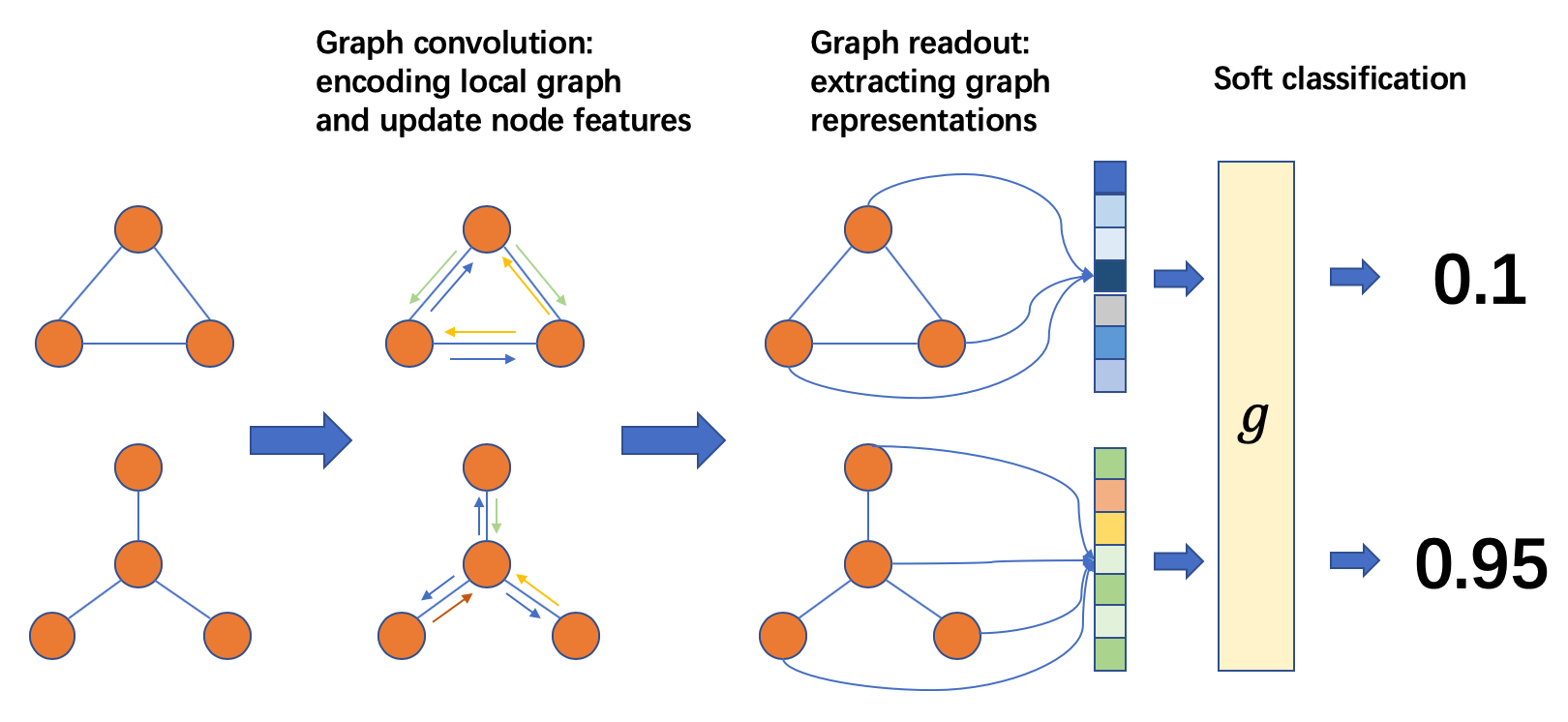From a batch of graphs, perform message passing and graph convolution for nodes to communicate with others. After message passing, compute a tensor for graph representation from node (and edge) attributes. This step might be called readout or aggregation. Finally, the graph representations are fed into a classifier $$g$$ to predict the graph labels.

Graph convolution layer can be found in the dgl.nn.<backend> submodule.

from dgl.nn.pytorch import GraphConv


For this demonstration, consider initial node features to be their degrees. After two rounds of graph convolution, perform a graph readout by averaging over all node features for each graph in the batch.

$h_g=\frac{1}{|\mathcal{V}|}\sum_{v\in\mathcal{V}}h_{v}$

In DGL, dgl.mean_nodes() handles this task for a batch of graphs with variable size. You then feed the graph representations into a classifier with one linear layer to obtain pre-softmax logits.

import torch.nn as nn
import torch.nn.functional as F

class Classifier(nn.Module):
def __init__(self, in_dim, hidden_dim, n_classes):
super(Classifier, self).__init__()
self.conv1 = GraphConv(in_dim, hidden_dim)
self.conv2 = GraphConv(hidden_dim, hidden_dim)
self.classify = nn.Linear(hidden_dim, n_classes)

def forward(self, g):
# Use node degree as the initial node feature. For undirected graphs, the in-degree
# is the same as the out_degree.
h = g.in_degrees().view(-1, 1).float()
# Perform graph convolution and activation function.
h = F.relu(self.conv1(g, h))
h = F.relu(self.conv2(g, h))
g.ndata['h'] = h
# Calculate graph representation by averaging all the node representations.
hg = dgl.mean_nodes(g, 'h')
return self.classify(hg)


## Setup and training¶

Create a synthetic dataset of $$400$$ graphs with $$10$$ ~ $$20$$ nodes. $$320$$ graphs constitute a training set and $$80$$ graphs constitute a test set.

import torch.optim as optim

# Create training and test sets.
trainset = MiniGCDataset(320, 10, 20)
testset = MiniGCDataset(80, 10, 20)
# Use DGL's GraphDataLoader. It by default handles the
# graph batching operation for every mini-batch.

# Create model
model = Classifier(1, 256, trainset.num_classes)
loss_func = nn.CrossEntropyLoss()
model.train()

epoch_losses = []
for epoch in range(80):
epoch_loss = 0
for iter, (bg, label) in enumerate(data_loader):
prediction = model(bg)
loss = loss_func(prediction, label)
loss.backward()
optimizer.step()
epoch_loss += loss.detach().item()
epoch_loss /= (iter + 1)
print('Epoch {}, loss {:.4f}'.format(epoch, epoch_loss))
epoch_losses.append(epoch_loss)


Out:

Epoch 0, loss 2.0216
Epoch 1, loss 1.9677
Epoch 2, loss 1.9630
Epoch 3, loss 1.9551
Epoch 4, loss 1.9381
Epoch 5, loss 1.9324
Epoch 6, loss 1.9154
Epoch 7, loss 1.8965
Epoch 8, loss 1.8759
Epoch 9, loss 1.8430
Epoch 10, loss 1.8069
Epoch 11, loss 1.7907
Epoch 12, loss 1.7345
Epoch 13, loss 1.6850
Epoch 14, loss 1.6257
Epoch 15, loss 1.5742
Epoch 16, loss 1.5157
Epoch 17, loss 1.4594
Epoch 18, loss 1.3970
Epoch 19, loss 1.3446
Epoch 20, loss 1.2999
Epoch 21, loss 1.2387
Epoch 22, loss 1.1933
Epoch 23, loss 1.1539
Epoch 24, loss 1.1009
Epoch 25, loss 1.0694
Epoch 26, loss 1.0542
Epoch 27, loss 1.0333
Epoch 28, loss 0.9920
Epoch 29, loss 0.9774
Epoch 30, loss 0.9403
Epoch 31, loss 0.9273
Epoch 32, loss 0.9032
Epoch 33, loss 0.8981
Epoch 34, loss 0.8737
Epoch 35, loss 0.8631
Epoch 36, loss 0.8469
Epoch 37, loss 0.8407
Epoch 38, loss 0.8392
Epoch 39, loss 0.8258
Epoch 40, loss 0.8213
Epoch 41, loss 0.7983
Epoch 42, loss 0.7891
Epoch 43, loss 0.7928
Epoch 44, loss 0.7761
Epoch 45, loss 0.7810
Epoch 46, loss 0.7894
Epoch 47, loss 0.7700
Epoch 48, loss 0.7714
Epoch 49, loss 0.7477
Epoch 50, loss 0.7656
Epoch 51, loss 0.7486
Epoch 52, loss 0.7510
Epoch 53, loss 0.7346
Epoch 54, loss 0.7543
Epoch 55, loss 0.7520
Epoch 56, loss 0.7163
Epoch 57, loss 0.7175
Epoch 58, loss 0.7083
Epoch 59, loss 0.7228
Epoch 60, loss 0.7320
Epoch 61, loss 0.7068
Epoch 62, loss 0.7070
Epoch 63, loss 0.7414
Epoch 64, loss 0.7157
Epoch 65, loss 0.7098
Epoch 66, loss 0.6850
Epoch 67, loss 0.6859
Epoch 68, loss 0.6896
Epoch 69, loss 0.6755
Epoch 70, loss 0.6898
Epoch 71, loss 0.6934
Epoch 72, loss 0.6721
Epoch 73, loss 0.6788
Epoch 74, loss 0.6686
Epoch 75, loss 0.6750
Epoch 76, loss 0.6761
Epoch 77, loss 0.6588
Epoch 78, loss 0.6454
Epoch 79, loss 0.6456


The learning curve of a run is presented below.

plt.title('cross entropy averaged over minibatches')
plt.plot(epoch_losses)
plt.show()The trained model is evaluated on the test set created. To deploy the tutorial, restrict the running time to get a higher accuracy ($$80$$ % ~ $$90$$ %) than the ones printed below.

model.eval()
# Convert a list of tuples to two lists
test_X, test_Y = map(list, zip(*testset))
test_bg = dgl.batch(test_X)
test_Y = torch.tensor(test_Y).float().view(-1, 1)
probs_Y = torch.softmax(model(test_bg), 1)
sampled_Y = torch.multinomial(probs_Y, 1)
argmax_Y = torch.max(probs_Y, 1).view(-1, 1)
print('Accuracy of sampled predictions on the test set: {:.4f}%'.format(
(test_Y == sampled_Y.float()).sum().item() / len(test_Y) * 100))
print('Accuracy of argmax predictions on the test set: {:4f}%'.format(
(test_Y == argmax_Y.float()).sum().item() / len(test_Y) * 100))


Out:

Accuracy of sampled predictions on the test set: 61.2500%
Accuracy of argmax predictions on the test set: 67.500000%


The animation here plots the probability that a trained model predicts the correct graph type.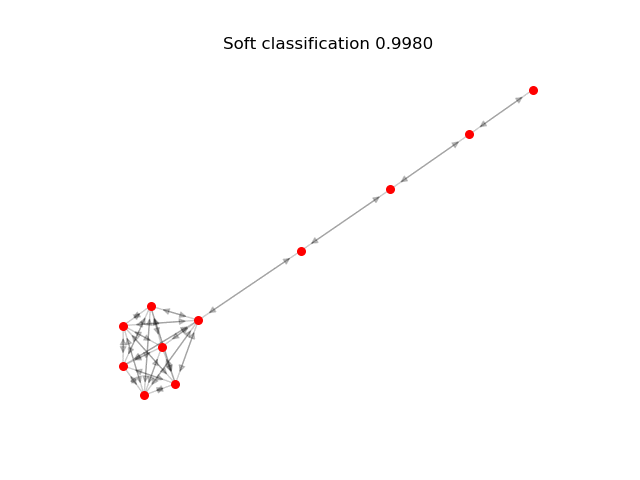To understand the node and graph representations that a trained model learned, we use t-SNE, for dimensionality reduction and visualization.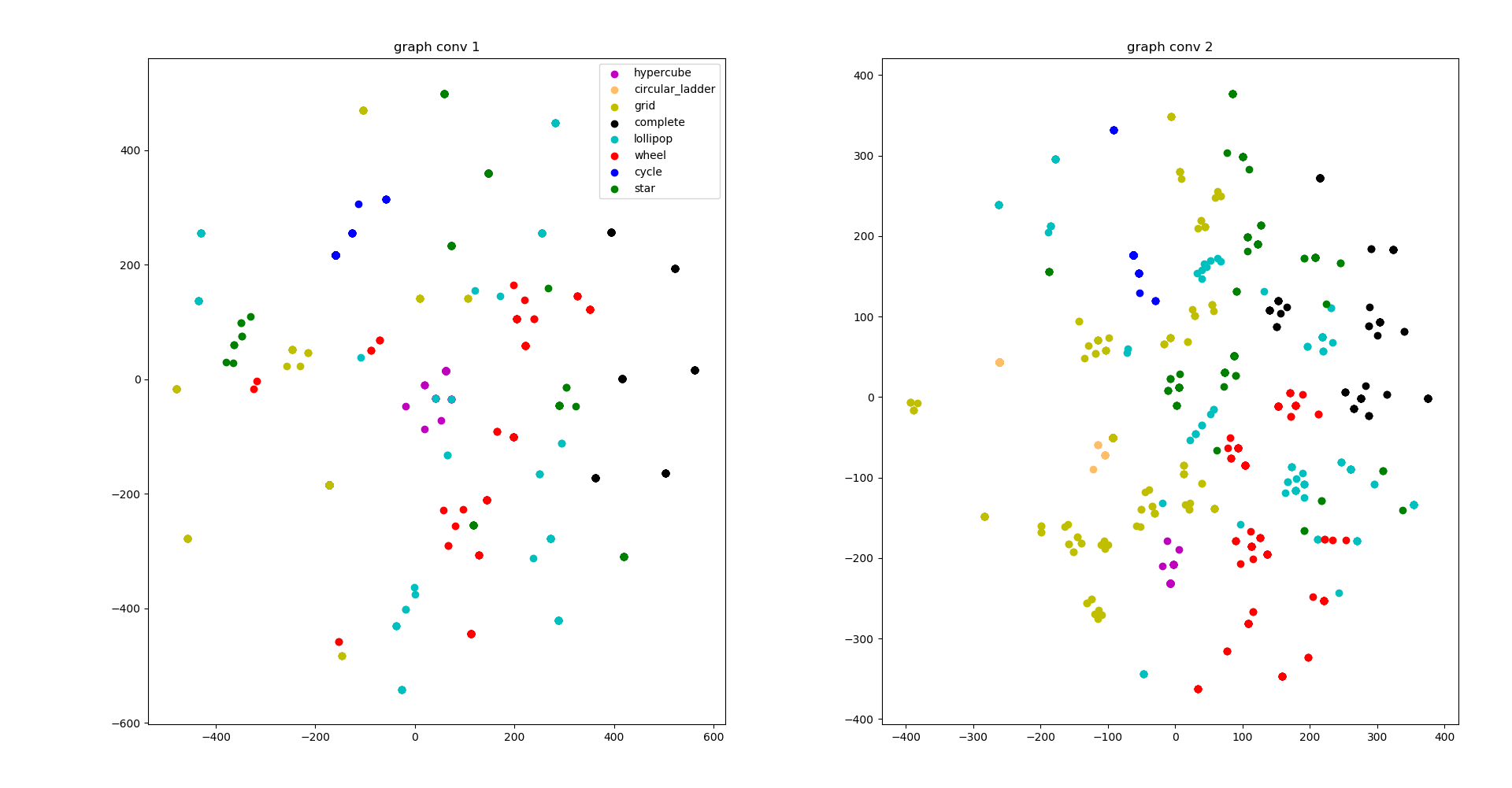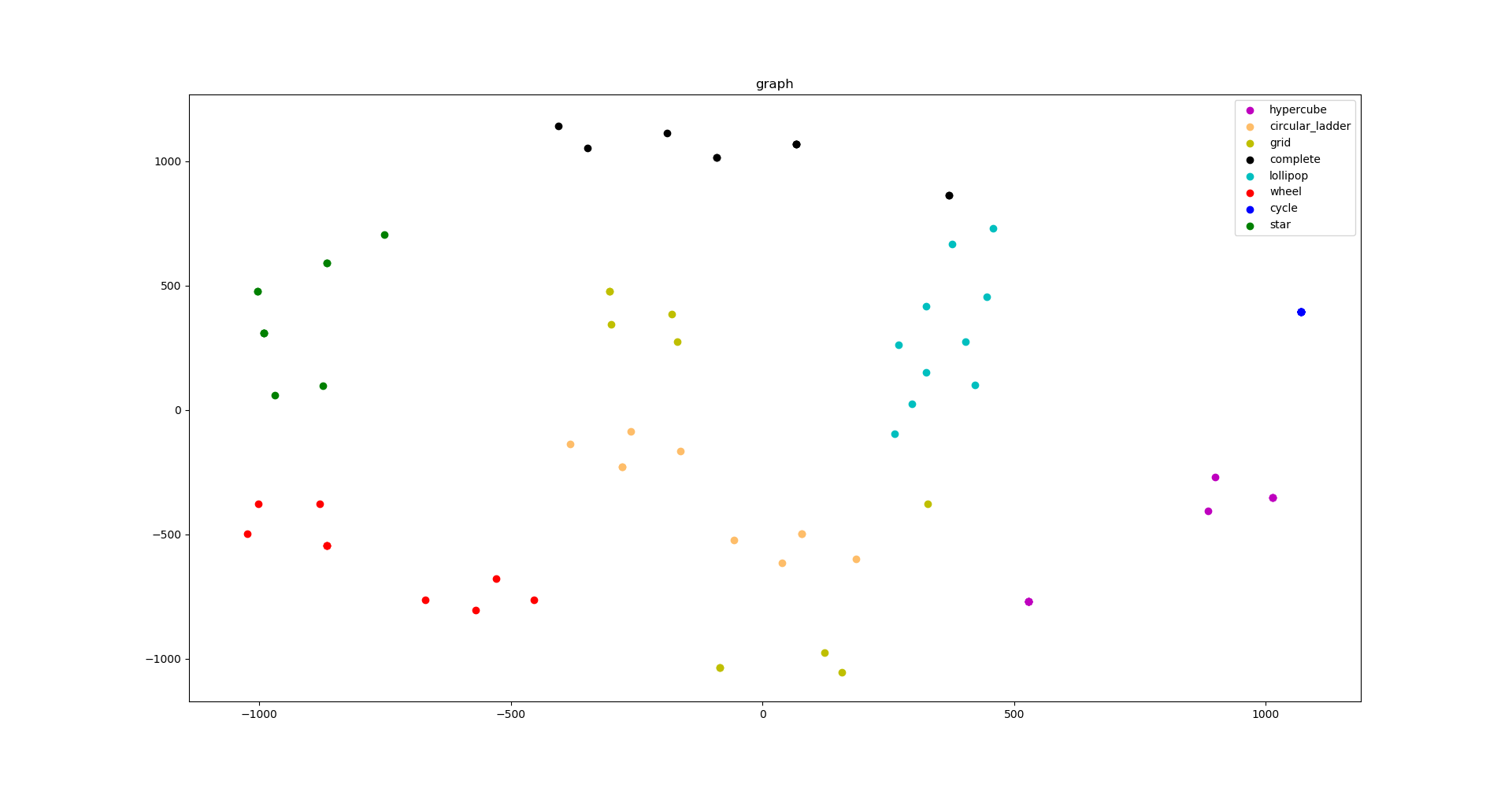The two small figures on the top separately visualize node representations after one and two layers of graph convolution. The figure on the bottom visualizes the pre-softmax logits for graphs as graph representations.

While the visualization does suggest some clustering effects of the node features, you would not expect a perfect result. Node degrees are deterministic for these node features. The graph features are improved when separated.

## What’s next?¶

Graph classification with graph neural networks is still a new field. It’s waiting for people to bring more exciting discoveries. The work requires mapping different graphs to different embeddings, while preserving their structural similarity in the embedding space. To learn more about it, see How Powerful Are Graph Neural Networks? a research paper published for the International Conference on Learning Representations 2019.

For more examples about batched graph processing, see the following:

Total running time of the script: ( 0 minutes 11.355 seconds)

Gallery generated by Sphinx-Gallery